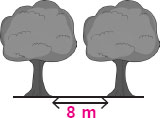# User Forum

Subject :IMO    Class : Class 3

Shreya planted some trees along one side of a fence. Each tree was planted 8 m apart. If the distance between the first tree and the last tree was 136 m, how many trees did she plant?A 17
B 18
C 16
D 19

Class : Class 4
Ans should B 18

Class : Class 3

Class : Class 5

Class : Class 4

Class : Class 4
[a] 17

Class : Class 3

## Ans 7:

Class : Class 5
ans b because we divide 136 by 8 , we get 17 and we have add 1 more as first tree...

Class : Class 3

Class : Class 3
17

Class : Class 3
(A) 17

## Ans 11:

Class : Class 4
it is not a - 17 it is b 18

Class : Class 4
whats the ans

Class : Class 3

Class : Class 3
136Ã·8Â±1=18

Class : Class 3

Class : Class 3

Class : Class 4
A

## Ans 18:

Class : Class 3
we divide 136 by 8 and then add one because the distance is between the first tree and the last tree so we have to calculate the first tree also

Class : Class 3
ans-18

Class : Class 3

## Ans 21:

Class : Class 3
Ans-(b) because if we divide 136 by 8 the answer would be 17 and add the first tree it would be 18

## Ans 22:

Class : Class 1
Aarav Tanwar from D.P.S. noida, class 3. A-17 is correct

## Ans 23:

Class : Class 4
B ,136 divided by 8 = 17 first tree =18.So ,answer is B

## Ans 24:

Class : Class 4
b can not be the ans because in the qes they have written that both the numbers are even but in ans b one smile is odd .

Class : Class 4
A-17

## Ans 26:

Class : Class 3
I think answer should be A.

## Ans 27:

Class : Class 4
Answer should A because when we divide 136 by 8,we get 17.First thing we won't add 1 tree more. So answer is A.

Class : Class 3